Test: Transportation Engineering- 1

# Test: Transportation Engineering- 1

Test Description

## 10 Questions MCQ Test GATE Civil Engineering (CE) 2023 Mock Test Series | Test: Transportation Engineering- 1

Test: Transportation Engineering- 1 for Civil Engineering (CE) 2023 is part of GATE Civil Engineering (CE) 2023 Mock Test Series preparation. The Test: Transportation Engineering- 1 questions and answers have been prepared according to the Civil Engineering (CE) exam syllabus.The Test: Transportation Engineering- 1 MCQs are made for Civil Engineering (CE) 2023 Exam. Find important definitions, questions, notes, meanings, examples, exercises, MCQs and online tests for Test: Transportation Engineering- 1 below.
Solutions of Test: Transportation Engineering- 1 questions in English are available as part of our GATE Civil Engineering (CE) 2023 Mock Test Series for Civil Engineering (CE) & Test: Transportation Engineering- 1 solutions in Hindi for GATE Civil Engineering (CE) 2023 Mock Test Series course. Download more important topics, notes, lectures and mock test series for Civil Engineering (CE) Exam by signing up for free. Attempt Test: Transportation Engineering- 1 | 10 questions in 30 minutes | Mock test for Civil Engineering (CE) preparation | Free important questions MCQ to study GATE Civil Engineering (CE) 2023 Mock Test Series for Civil Engineering (CE) Exam | Download free PDF with solutions
 1 Crore+ students have signed up on EduRev. Have you?
Test: Transportation Engineering- 1 - Question 1

### A super-elevation e is provided on a circular horizontal curve such that a vehicle can be stopped on the curve without sliding. Assuming a design speed v and maximum coefficient of side friction fmax, which one of the following criteria should be satisfied?

Detailed Solution for Test: Transportation Engineering- 1 - Question 1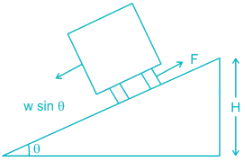Let the weight of vehicle = W in stopping condition the friction force F

should be greater than w sin θ to prevent the sliding F > w sin θ

for smaller value of Q

tan θ = e = sin θ

F = w × f

w × f ≥ w × e

f > e

Test: Transportation Engineering- 1 - Question 2

### Which of the following statements CANNOT be used to describe free-flow speed (uf) of a traffic stream?

Detailed Solution for Test: Transportation Engineering- 1 - Question 2

Free flow speed (Vf) is that speed at which flow and density is negligible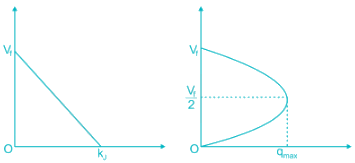Test: Transportation Engineering- 1 - Question 3

### The safety within a roundabout and the efficiency of a roundabout can be increased respectively by

Detailed Solution for Test: Transportation Engineering- 1 - Question 3

The essence of the intersection control is to resolve these conflicts at the intersection for the safe and efficient movement of both vehicular traffic and pedestrians.

The safety within a roundabout and the efficiency of a roundabout can be increased respectively by decreasing the entry radius and increasing the exit radius.

Note:

There are two methods of intersection controls

1) time sharing and

2) space sharing.

The type of intersection control that has to be adopted depends on the traffic volume, road geometry, cost involved, importance of the road etc.

*Answer can only contain numeric values
Test: Transportation Engineering- 1 - Question 4

While aligning a hill road with a ruling gradient of 6%, a horizontal curve of radius 50 m is encountered. The grade compensation (in percentage up two equal places) to be provided for this case would be _______

Detailed Solution for Test: Transportation Engineering- 1 - Question 4

R = 50

Grade compensation =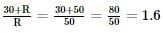(Grade compensation)max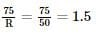Test: Transportation Engineering- 1 - Question 5

If the total number of commercial vehicles per day ranges from 3000 to 6000, the minimum percentage of commercial traffic to be surveyed for axle load is:

Detailed Solution for Test: Transportation Engineering- 1 - Question 5

Minimum percentage is 15% for axle load determination when commercial vehicles vary from 3000-6000.

Sample size for axle load survey: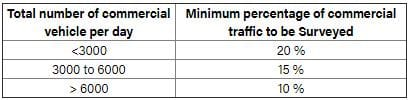*Answer can only contain numeric values
Test: Transportation Engineering- 1 - Question 6

The VPI (vertical point of intersection) is 100 m away (when measured along the horizontal) from the VPC (vertical point of curvature). If the vertical curve is parabolic the length of the curve (in meters and measured along the horizontal) is ________

Detailed Solution for Test: Transportation Engineering- 1 - Question 6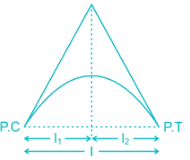For flat vertical curve we assume that l= l2

l = l1 + l2 = 100 + 100 = 200 m

Test: Transportation Engineering- 1 - Question 7

A circular curve of radius R connects two straights with a deflection angle of 60°. The tangent length is

Detailed Solution for Test: Transportation Engineering- 1 - Question 7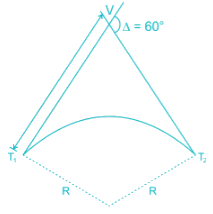Tangent length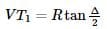= R tan 30° = 0.577 R

Test: Transportation Engineering- 1 - Question 8

On a circular curve, the rate of super-elevation is e. While negotiating the curve a vehicle comes to a stop. It is seen that the stopped vehicle does not slide inwards (in the radial direction). The coefficient of side friction is f. Which of the following is true:

Detailed Solution for Test: Transportation Engineering- 1 - Question 8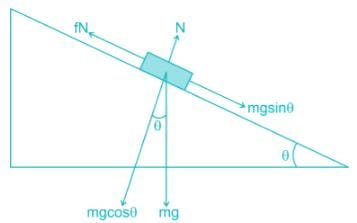For equilibrium

fN ≥ mg sin θ

⇒ fmg cos θ ≥ mg sin θ

⇒ fcos θ ≥ sin θ

⇒ f ≥ tan θ

f ≥ e

*Answer can only contain numeric values
Test: Transportation Engineering- 1 - Question 9

In a one-lane one-way homogeneous traffic stream, the observed average headway is 3.0 s. The flow (expressed in vehicles/hr) in this traffic stream is________

Detailed Solution for Test: Transportation Engineering- 1 - Question 9

Flow of traffic stream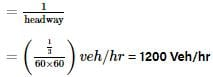Test: Transportation Engineering- 1 - Question 10

A two-lane, one-way road with a radius of 50 m is predominantly carrying lorries with a wheelbase of 5 m. The speed of lorries is restricted to be between 60 kmph and 80 kmph. The mechanical widening and psychological widening required at 60 kmph are designated as wme,60 and wps,60, respectively. The mechanical widening and psychological widening required at 80 kmph are designated as wme,80 and wps,80, respectively. The correct values of wme,60, wps,60, wme,80, wps,80, respectively are

Detailed Solution for Test: Transportation Engineering- 1 - Question 10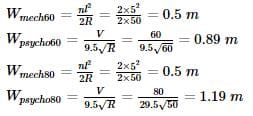## GATE Civil Engineering (CE) 2023 Mock Test Series

27 docs|296 tests
 Use Code STAYHOME200 and get INR 200 additional OFF Use Coupon Code
Information about Test: Transportation Engineering- 1 Page
In this test you can find the Exam questions for Test: Transportation Engineering- 1 solved & explained in the simplest way possible. Besides giving Questions and answers for Test: Transportation Engineering- 1, EduRev gives you an ample number of Online tests for practice

## GATE Civil Engineering (CE) 2023 Mock Test Series

27 docs|296 tests